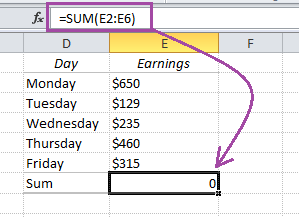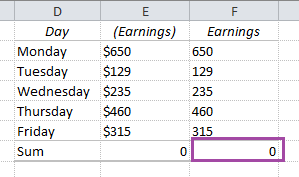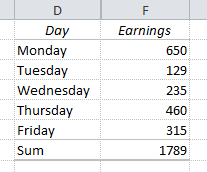# Convert text to numbers in Excel using formulasHave you ever imported data into Excel that won’t calculate? Look at this example where the earnings look like numbers to the human eye, but not to Excel. And when Excel is not able to identify any numbers, it can’t calculate any sums or averages either.

This happens all the time if you use data from other data sources: It could be a txt-file from a booking system, a copy-paste from a website or even a database export.

Enough about the problem. Let’s solve it, and let’s not spend more than 2 minutes doing it!

## 1. Get rid of the textTo isolate the numbers we should get rid of the dollar sign in front of them. There are several ways to do it – I’ll mention a few of them:

a) The RIGHT function: =RIGHT(E2,3)

This function returns the 3 rightmost characters in cell E2. This works as long as we only have 3-digit numbers, but if any of the records are more or less than that, we’re in trouble.

b) Combine RIGHT and LEN: =RIGHT(E2,LEN(E2)-1)

Instead of the static number 3 in the first function we use the LEN function to count characters. I have replaced the number 3 with the function LEN(E2)-1 inside the RIGHT function, so now the RIGHT function will always return the correct number of characters (total number of characters minus one).

c) The REPLACE function: =REPLACE(E2,1,1,””)

There are 4 arguments in this function: old_text (E2), start_num (1), num_chars (1) and new_text (“”). In English: The first character in E2 is \$, and this formula replaces the dollar sign with “”, which means nothing (a null-string).

I’ll use the RIGHT and LEN combo, which removes the dollar signs as expected, but the sum is still zero!

That’s because Excel still believes that the numbers are text strings!

## 2. Convert text to numberTo convert a number stored as text to a number, just add 0! In our example =RIGHT(E2,LEN(E2)-1) becomes =RIGHT(E2,LEN(E2)-1)+0.

It works, and we can hide (not delete!) the column with the original data.

Note: If you use comma as the decimal separator as default (applies to most non-English users) you have to replace the commas in the formulas with semicolons.

Are you using a non-English version of Excel? Click here for translations of the 100 most common functions.

## 1 thought on “Convert text to numbers in Excel using formulas”

1.Yunus on said:

=RIGHT(E2,LEN(E2)-1)+0. The formula gives error in Excel 2013, any solutions ?